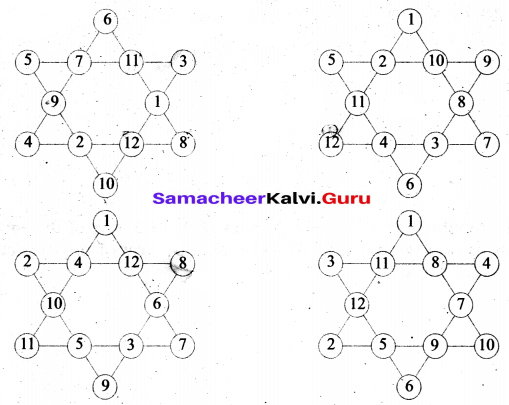# Samacheer Kalvi 6th Maths Solutions Term 1 Chapter 6 Information Processing Ex 6.2## Tamilnadu Samacheer Kalvi 6th Maths Solutions Term 1 Chapter 6 Information Processing Ex 6.2

Question 1.
In the following magic triangle, arrange the numbers from 1 to 6, so that you get the same sum on all its sides.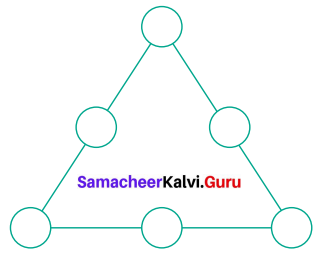Solution:
Step 1: Complete the corners with smaller numbers 1, 2 and 3.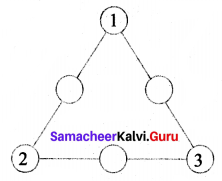Step 2: The side having smallest numbers 1 & 2 are to be filled with the greatest number 6, the second smallest 1 & 3 side to be filled with the second largest 5 at the middle and so on.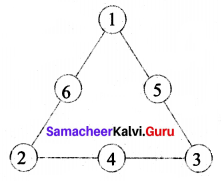The magic sum is 1 + 6 + 2 = 2 + 4 + 3 = 3 + 5 + 1 = 9. Some other ways are given below.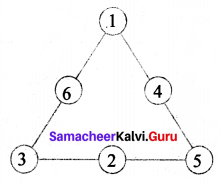The magic sum = 1 + 6 + 3 = 3 + 2 + 5 = 5 + 4 + 1 = 10.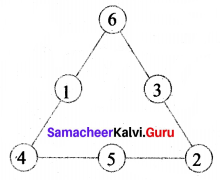The magic sum 6 + 1 + 4 = 4 + 5 + 2 = 2 + 3 + 6 = 11.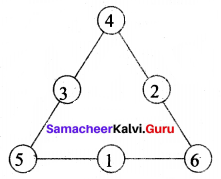The magic sum 4 + 3 + 5 = 5 + 1 + 6 = 6 + 2 + 4 = 12.

Question 2.
Using the numbers from 1 to 9
(i) Can you form a magic triangle?
(ii) How many magic triangles can be formed?
(iii) What are the sums of the sides of the magic triangle?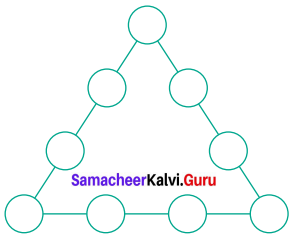Solution:
(i) Yes, we can form
(ii) 5
(iii)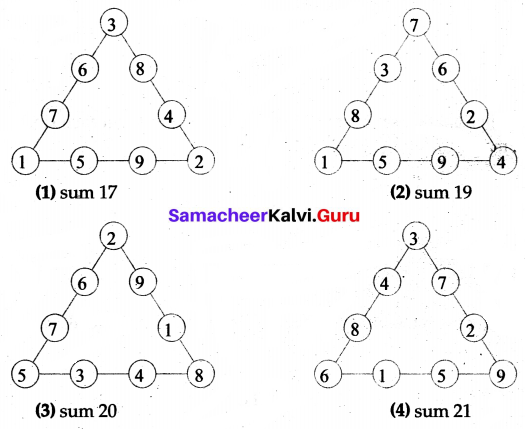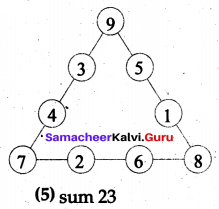Sums are 17, 19, 20, 21 and 23.Question 3.
Arrange the odd numbers from 1 to 17 without repetition to get a sum of 30 on each side of the magic triangle.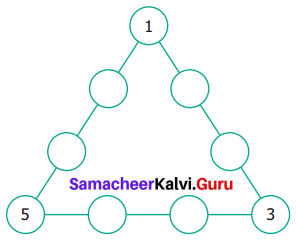Solution:
The odd numbers between 1 to 17 are 1, 3, 5,7,9,11,13,15,17.
Step 1: Place the smaller numbers 1, 3, 5 on the comers.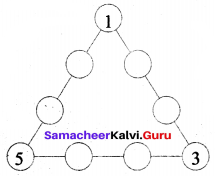Step 2: Arrange another set of smaller numbers 7, 9 and 11 on each side.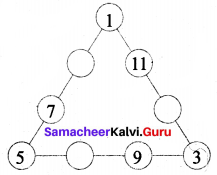Step 3: Arrange the remaining numbers 13,15,17 to give the total 30.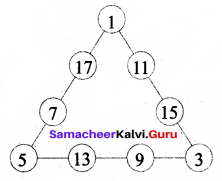Magic sum = 30.

Question 4.
Put the numbers 1, 2, 3, 4, 5, 6 and 7 in the circles so that each straight line of three numbers add up to the same total.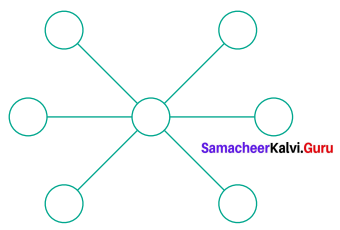Solution:
Here there is even number of terms. Also we know that 1 + 6 = 7, 2 + 5 = 7, 3 + 4 = 7; so placing 7 at the centre, and the pairs (1, 6) (2, 5) and (3, 4) at. the opposite ends we get, the answer.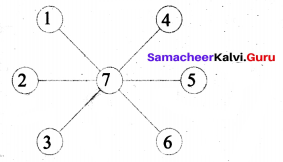Question 5.
Place the number 1 to 12 in the 12 circles so that the sum of the numbers in each of the six lines of the star is 26. Use each number from 1 to 12 exactly once. Find more possible ways.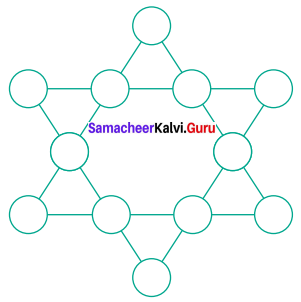Solution:
The given star can be viewed as two magical triangular as.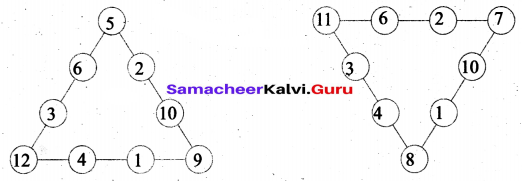Now the required arrangement is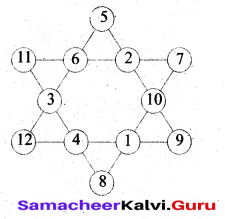Some other arrangements are